Publicité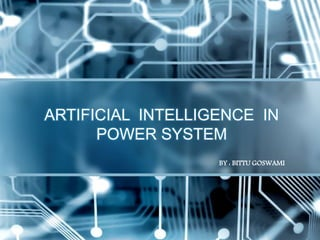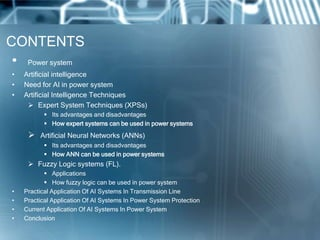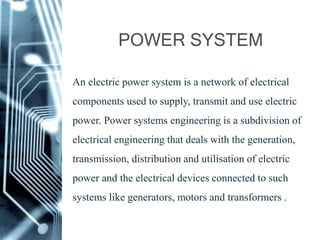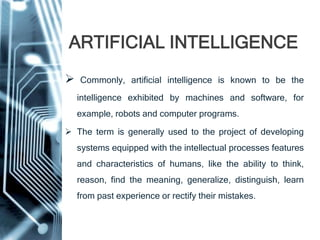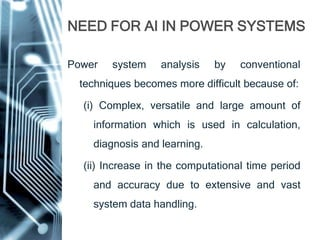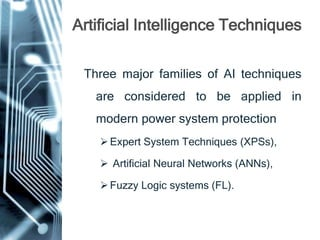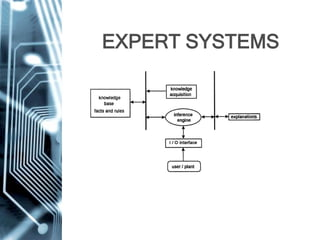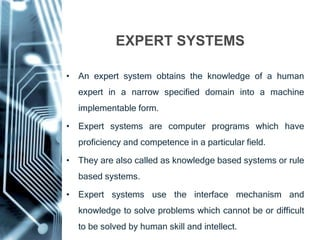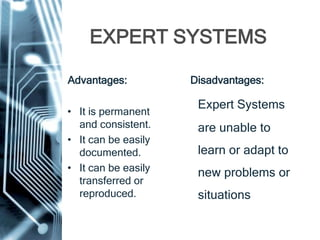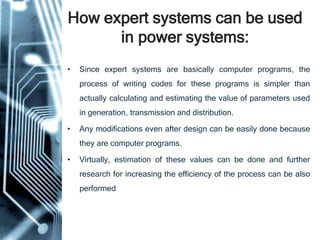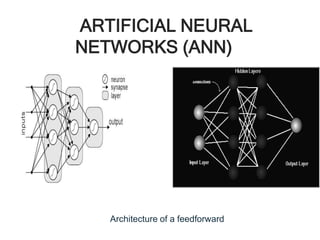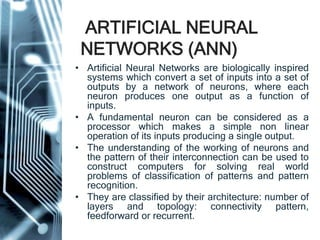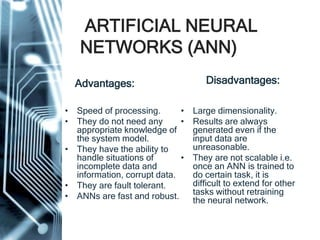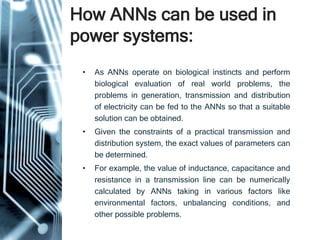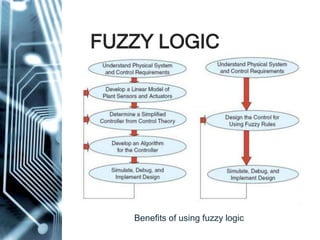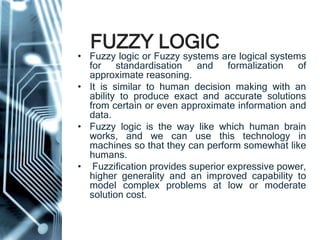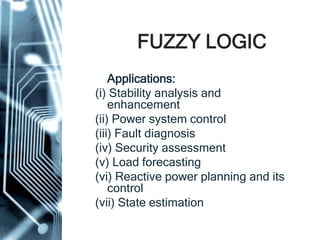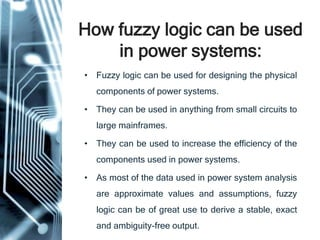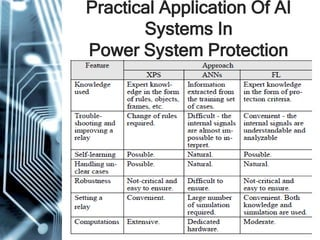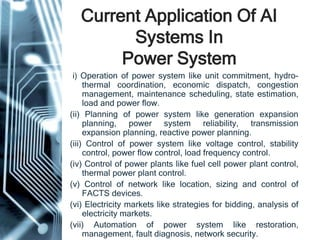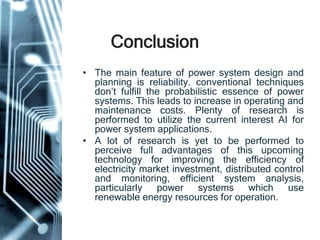1 sur 24
Publicité

### Artificial intelligence in power system

1. ARTIFICIAL INTELLIGENCE IN POWER SYSTEM BY : BITTU GOSWAMI
2. CONTENTS • Power system • Artificial intelligence • Need for AI in power system • Artificial Intelligence Techniques  Expert System Techniques (XPSs)  Its advantages and disadvantages  How expert systems can be used in power systems  Artificial Neural Networks (ANNs)  Its advantages and disadvantages  How ANN can be used in power systems  Fuzzy Logic systems (FL).  Applications  How fuzzy logic can be used in power system • Practical Application Of AI Systems In Transmission Line • Practical Application Of AI Systems In Power System Protection • Current Application Of AI Systems In Power System • Conclusion
3. POWER SYSTEM An electric power system is a network of electrical components used to supply, transmit and use electric power. Power systems engineering is a subdivision of electrical engineering that deals with the generation, transmission, distribution and utilisation of electric power and the electrical devices connected to such systems like generators, motors and transformers .
4. ARTIFICIAL INTELLIGENCE  Commonly, artificial intelligence is known to be the intelligence exhibited by machines and software, for example, robots and computer programs.  The term is generally used to the project of developing systems equipped with the intellectual processes features and characteristics of humans, like the ability to think, reason, find the meaning, generalize, distinguish, learn from past experience or rectify their mistakes.
5. NEED FOR AI IN POWER SYSTEMS Power system analysis by conventional techniques becomes more difficult because of: (i) Complex, versatile and large amount of information which is used in calculation, diagnosis and learning. (ii) Increase in the computational time period and accuracy due to extensive and vast system data handling.
6. Artificial Intelligence Techniques Three major families of AI techniques are considered to be applied in modern power system protection Expert System Techniques (XPSs),  Artificial Neural Networks (ANNs), Fuzzy Logic systems (FL).
7. EXPERT SYSTEMS
8. • An expert system obtains the knowledge of a human expert in a narrow specified domain into a machine implementable form. • Expert systems are computer programs which have proficiency and competence in a particular field. • They are also called as knowledge based systems or rule based systems. • Expert systems use the interface mechanism and knowledge to solve problems which cannot be or difficult to be solved by human skill and intellect. EXPERT SYSTEMS
9. EXPERT SYSTEMS Advantages: • It is permanent and consistent. • It can be easily documented. • It can be easily transferred or reproduced. Disadvantages: Expert Systems are unable to learn or adapt to new problems or situations.
10. How expert systems can be used in power systems: • Since expert systems are basically computer programs, the process of writing codes for these programs is simpler than actually calculating and estimating the value of parameters used in generation, transmission and distribution. • Any modifications even after design can be easily done because they are computer programs. • Virtually, estimation of these values can be done and further research for increasing the efficiency of the process can be also performed.
11. ARTIFICIAL NEURAL NETWORKS (ANN) Architecture of a feedforward ANN Typical structure of an ANN
12. ARTIFICIAL NEURAL NETWORKS (ANN) • Artificial Neural Networks are biologically inspired systems which convert a set of inputs into a set of outputs by a network of neurons, where each neuron produces one output as a function of inputs. • A fundamental neuron can be considered as a processor which makes a simple non linear operation of its inputs producing a single output. • The understanding of the working of neurons and the pattern of their interconnection can be used to construct computers for solving real world problems of classification of patterns and pattern recognition. • They are classified by their architecture: number of layers and topology: connectivity pattern, feedforward or recurrent.
13. ARTIFICIAL NEURAL NETWORKS (ANN) Advantages: • Speed of processing. • They do not need any appropriate knowledge of the system model. • They have the ability to handle situations of incomplete data and information, corrupt data. • They are fault tolerant. • ANNs are fast and robust. Disadvantages: • Large dimensionality. • Results are always generated even if the input data are unreasonable. • They are not scalable i.e. once an ANN is trained to do certain task, it is difficult to extend for other tasks without retraining the neural network.
14. How ANNs can be used in power systems: • As ANNs operate on biological instincts and perform biological evaluation of real world problems, the problems in generation, transmission and distribution of electricity can be fed to the ANNs so that a suitable solution can be obtained. • Given the constraints of a practical transmission and distribution system, the exact values of parameters can be determined. • For example, the value of inductance, capacitance and resistance in a transmission line can be numerically calculated by ANNs taking in various factors like environmental factors, unbalancing conditions, and other possible problems.
15. FUZZY LOGIC Benefits of using fuzzy logic
16. FUZZY LOGIC • Fuzzy logic or Fuzzy systems are logical systems for standardisation and formalization of approximate reasoning. • It is similar to human decision making with an ability to produce exact and accurate solutions from certain or even approximate information and data. • Fuzzy logic is the way like which human brain works, and we can use this technology in machines so that they can perform somewhat like humans. • Fuzzification provides superior expressive power, higher generality and an improved capability to model complex problems at low or moderate solution cost.
17. FUZZY LOGIC Applications: (i) Stability analysis and enhancement (ii) Power system control (iii) Fault diagnosis (iv) Security assessment (v) Load forecasting (vi) Reactive power planning and its control (vii) State estimation
18. How fuzzy logic can be used in power systems: • Fuzzy logic can be used for designing the physical components of power systems. • They can be used in anything from small circuits to large mainframes. • They can be used to increase the efficiency of the components used in power systems. • As most of the data used in power system analysis are approximate values and assumptions, fuzzy logic can be of great use to derive a stable, exact and ambiguity-free output.
19. Practical Application Of AI Systems In Transmission Line
20. Practical Application Of AI Systems In Transmission Line • If any fault occurs in the transmission line, the fault detector detects the fault and feeds it to the fuzzy system. Only three line currents are sufficient to implement this technique and the angular difference between fault and pre-fault current phasors are used as inputs to the fuzzy system. Fuzzy systems can be generally used for fault diagnosis. • Artificial Neural Networks and Expert systems can be used to improve the performance of the line. The environmental sensors sense the environmental and atmospheric conditions and give them as input to the expert systems. The expert systems are computer programs which provide the value of line parameters to be deployed as the output.
21. Practical Application Of AI Systems In Power System Protection
22. Current Application Of AI Systems In Power System (i) Operation of power system like unit commitment, hydro- thermal coordination, economic dispatch, congestion management, maintenance scheduling, state estimation, load and power flow. (ii) Planning of power system like generation expansion planning, power system reliability, transmission expansion planning, reactive power planning. (iii) Control of power system like voltage control, stability control, power flow control, load frequency control. (iv) Control of power plants like fuel cell power plant control, thermal power plant control. (v) Control of network like location, sizing and control of FACTS devices. (vi) Electricity markets like strategies for bidding, analysis of electricity markets. (vii) Automation of power system like restoration, management, fault diagnosis, network security.
23. Conclusion • The main feature of power system design and planning is reliability. conventional techniques don’t fulfill the probabilistic essence of power systems. This leads to increase in operating and maintenance costs. Plenty of research is performed to utilize the current interest AI for power system applications. • A lot of research is yet to be performed to perceive full advantages of this upcoming technology for improving the efficiency of electricity market investment, distributed control and monitoring, efficient system analysis, particularly power systems which use renewable energy resources for operation.
Publicité